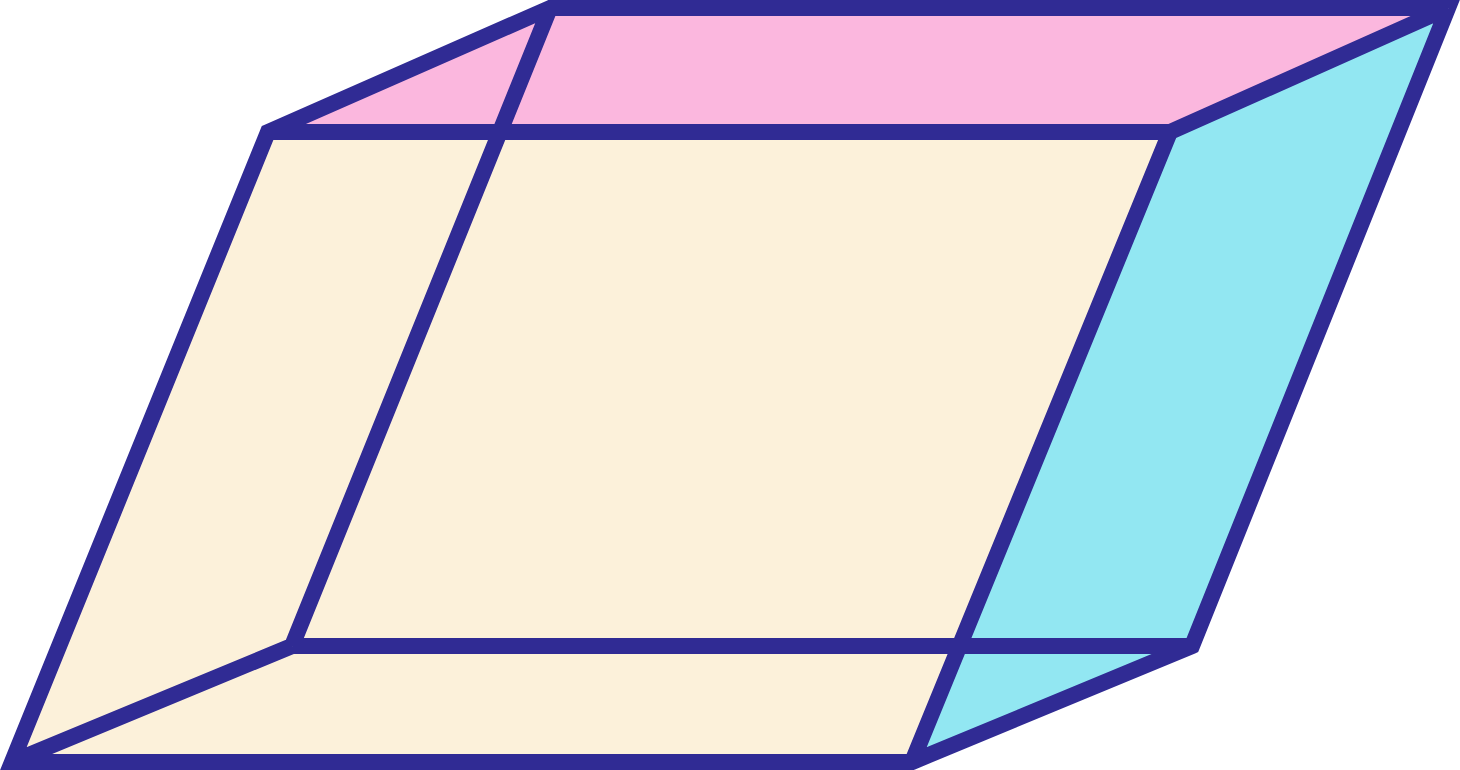# Maximized Parallelepiped

Algebra Level 3Let $\hat{u}$ and $\hat{v}$ be unit vectors and $\vec{w}$ be a vector such that $\vec{w}+(\vec{w}\ \times \hat{u})$ $=$ $\hat{v}$.

The angle in degrees between $\hat{u}$ and $\hat{v}$ such that $|(\hat{u} \times \hat{v}) \cdot \vec{w}|$ is maximized is $\theta$ and the maximum value of $|(\hat{u} \times \hat{v}) \cdot \vec{w}|$ is $M$. Find the value of $\theta + M$.

$Image$ $Credit :$ $Wikipedia$

×

Problem Loading...

Note Loading...

Set Loading...